# Problems on Trains with Solutions - PDF

### Some Important Points to Remember

#1
18 km/hour = 5 metre/second, can be used to convert the speed given in km/hour to metre/second or vice - versa.

3 km/hour = (3 × 5 / 18) metre/second

and

3 metre/second = (3 × 18 / 5) km/hour

#2
If a train of length "L" at a speed "v "passes another object of length "i" at a speed x in time "t", then
t = time to cross the object = Total Distance / Relative Speed = L + i / v - x
• If object is stationary, then x = 0
• If object is opposite, then put x = -x
• and v - x making after is (v + x)
A train is said to have crossed an object (stationary or moving) only when the last coach (end) of the train crosses the said object completely. It implies that the total length of the train has crossed the total length of the object.
Hence, the distance covered by the train = length of train + length of object

### Basic Formula

1. Time = Distance / Speed
2. Time to cross an object moving in the direction of train =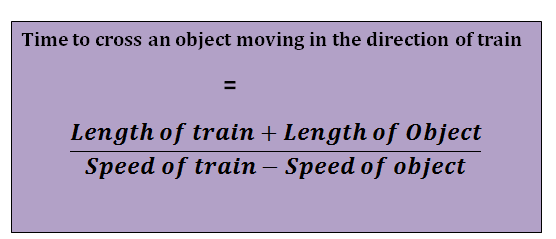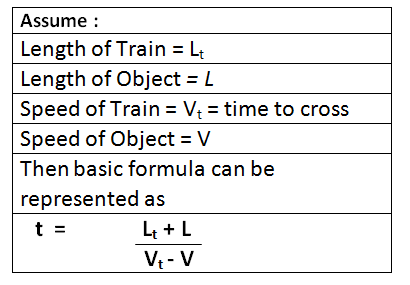### Important Note

1. If the object is of negligible length, then put length of object, L = 0 (i.e. tree, man)
2. If the object is stationary, then put speed of object, V = 0
3. If the object is moving in opposite direction, then put (-) ve sign before V, so the denominator of the formula becomes### Different Types of ObjectsIf the object is moving to opposite direction, then denominator becomes speed of (train + object)

### Two Trains Crossing Each Other in Both Directions

Two trains are crossing each other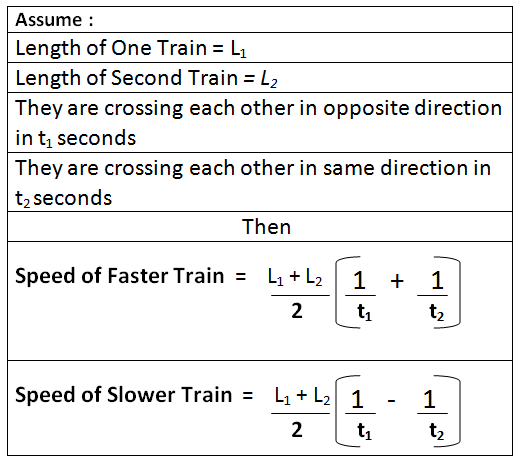APPLICATIONS ON TRAINS.

1.        How long will a train 130m long travelling at 40 km an hour take to pass a    kilometer stone?2.        How long will a train 60 m long travelling at 40 km an    hour take to pass through a station whose platform is 90 m  long?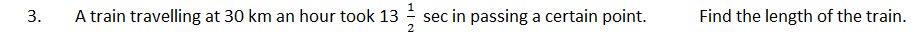4.        Find the length of a bridge which a train 130 m long, travelling at 45 km an  hour, can cross in 30 secs.5.        The length of the train that takes 8 seconds to pass a pole when it turns at a             speed of 36 km/hr is __________________ metres.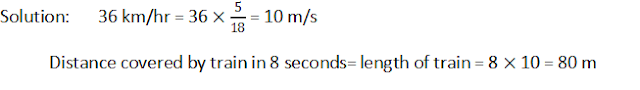6.        A train 50 metres long passes a platform 100 metres long I 10 seconds. The speed of the train is _____________ km/hr.7.        How many seconds will a train 60 m in length, travelling at the rate of 42      km an hour, take to pass another train 84 m long, proceeding in the same     direction at the rate of 30 km an hour?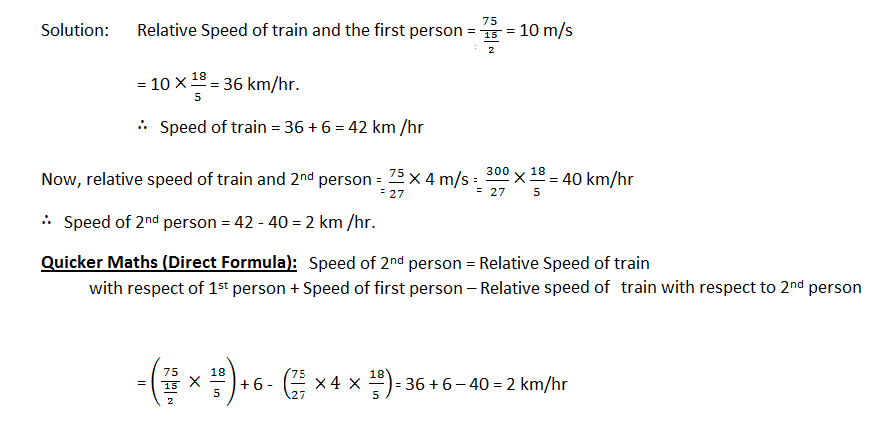9.        Two trains running at the rates 45 and 36 km an hour respectively, on           parallel rails in opposite directions, are observed to pass each other in 8             seconds, and when they are running in the same direction at the same rate         as before, a person sitting in the faster train observes that he passes the            other in 30 seconds. Find the lengths of the trains.10.      Two trains measuring 100 m and 80 m respectively, run on parallel lines of            rails. When travelling in opposite directions they are observed to pass each             other in 9 seconds, but when they are running in the same direction at the            rates as before, the faster train passes the other in 18 seconds. Find the          speed of the two trains in km per hour.#### What's trending in BankExamsToday

Smart Prep Kit for Banking Exams by Ramandeep Singh - Download here